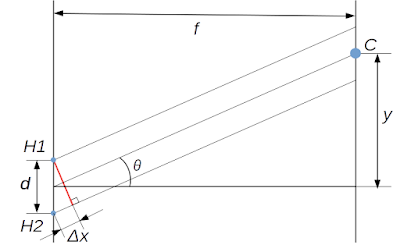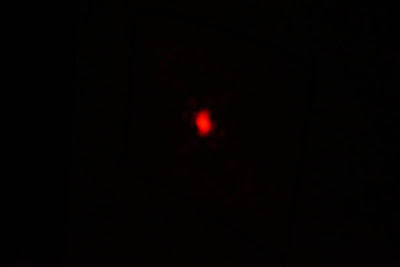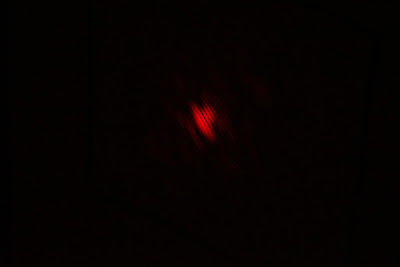## 2019-04-27

### Borissing

New word: Borissing

1. A behavior who started something, but succeeded to running away without taking responsibility.
Usage example: a school teacher told a student, "Please don't borissing. You are already a 5th grade."

2. Referring an social harassment. Some people coincidentally has a similar name. There are some incidents, such child was picked in a class. The association "We did nothing wrong" officially stated, this is a discrimination, please stop using the word. But another association, "Yes, but this is the consequence" stated recently, we should solve the problem from the source, otherwise we could not completely solve this problem.

-- in the Devil's dictionary draft

## 2019-02-05

### Young's double slit equation: Many textbooks draw parallel rays, but how could parallel rays intersect?

Young's double slit equation is well known result that shows the light has a wave property.
A typical illustration of Young's interference experiment is shown in Figure 1.  This figure confuses novice learners since at the point C, two parallel rays intersect. But how could possibly parallel rays intersect? In Euclidean geometry, parallel two lines will never intersect.Figure 1: Typical explanation figure: Two parallel rays and path length difference $\Delta x$Figure 2: Precise figure: Two rays intersect at point C to cause interference.
Figure 2 shows more precise figure of Young's interference experiment. At the point C, two rays meet and cause an interference depends on the path difference from the holes H1 and H2. When you zoom in to the two holes part, you see the precise path length difference $$\Delta x_r$$ is slightly differ from the $$\Delta x$$. The real path length difference $$\Delta x_r$$ is
$d \sin (\theta - \alpha) = \Delta x_r,$
where the distance between two slits is $$d$$.Figure 3: Precise figure: Two rays meet at point C.

Many explanations noted: The distance $$f$$ is enough large compare to the $$d$$.

This implies $$\alpha$$ is quite small. But I usually doesn't see this  hint, $$\alpha$$ is quite small''. I would like to see this hint.

If I see this hint about $$\alpha$$, then I could say, ok, $$\alpha$$ is small, then $$\cos \alpha \approx 1$$, $$\sin \alpha \approx 0$$. But I would like to say what is the order since this is also relative to $$\theta$$. And $$\theta$$ is usually relatively small, too. Why we don't say $$\sin \alpha \approx 0$$, $$\cos \theta \approx 0$$? If someone said so, then distance is always 0 and this figure doesn't make any sense. When I was a high school student, I had this problem.

One of the reason here is when $$f$$ is larger, actually $$\theta$$ doesn't change and $$\alpha$$ becomes smaller, so, I could figure out that
$\sin (\theta - \alpha) \approx \sin \theta.$
Maybe we should realize this by myself, but it is nice if the textbook tells that more directly instead of, we assume $$f$$ is enough large.

One more observation is Maclaurin expansion of $$\sin$$ and $$\cos$$.  If you learn calculus, this is simple, but for novice learner it is also nice to mention that.

When we expand the $$\sin (\theta - \alpha)$$,
$\sin (\theta - \alpha) = \sin \theta \cos \alpha - \cos \theta \sin \alpha.$
Now, consider the Maclaurin expansion to approximate the $$\sin x$$ and $$\cos x$$, just the first two teams are:
$\sin x \approx x - \frac{x^3}{3!}\\ \cos x \approx 1 - \frac{x^2}{2!}$
When $$x$$ is small, actually only the first term of $$\sin$$ and $$\cos$$  matters if they are multiplied. Since especially the second term of $\cos$ is much smaller than the $$\sin$$'s first term. $$\alpha$$ is so small, we can easily assume it is less than 1 degree, which is 0.017 rad. Even $$\theta$$ is 1 degree, 0.017 rad, the $$\cos$$ part became  0.00029. It is almost 100 times smaller than the $$\sin$$ term. And $$\alpha$$ can be much smaller.

Therefore, we can use Figure 1 for how to calculate the interference pattern.

It is useful, but I had a problem of the figure, since it seems two parallel rays meet at the point C. And parallel rays should not intersect.

After I learned calculus and how to approximate the $$\sin$$ and $$\cos$$, then this became clear, but I think it is nice to write this small article for the learners like high school student me.

Here is my experiment results.Figure 4: Set up (left one hole, middle and right two holes).Figure 5: One hole case (no interference)Figure 6: two holes (interference)

## 2018-12-27

### How to remove the video recording time limitation of a Sony camera (Alpha 5000)

I have a Sony camera (Sony Alpha 5000) and I like it. But I have a problem of limitation of video recording time: 30 minutes.

Today I found a way to remove this limitation. I saw the next video:

The video creator said, we can disable the limit for most of the Sony's camera. I only tested Sony Alpha 5000.

The procedure

Camera preparation

• Switch on the camera
• Menu -- Setup --- USB connection, set connection to MTP
• Switch off and on the camera
• Connect to a computer with USB cable (keep connecting)

Note: This site is from Sony, but the software is not from Sony, so no guarantee. you should use this your own risk. I can not take any responsibility.

• From the above URL, you can go to OpenMemories page

I once closed the page and open a page with https://sony-pmca.appspot.com/apps and went to OpenMemories again

• Now You see in the log
If you have not installed PMCADownloader yet, you don't see the Plugin loaded'' line. It might be better to restart your browser after installed the PMCADownloader.
• Push Get camera info'' to test the plugin is working. If it is working, you will see your camera information in a several seconds.
• Push Install OpenMeories: Tweak'' to install the tweak application to the camera
• Select the video tab, check the Disable video recording limit''
I tested to record, but around one hour, it shut down with a message like internal temperature is too high and cool down''. Still this error message actually helps me. Because the normal mode silently shutdown and I could not notice it.